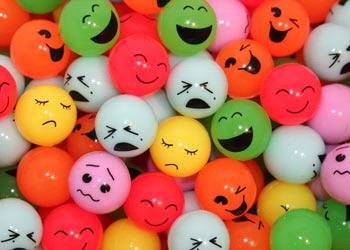# Balls And Bags

Probability Level 2You are given an bag filled with 20 red balls, 30 white balls and 40 blue balls. You decide to randomly take 15 balls out of the bag without replacement. What is the probability that the last ball you draw from the bag will be a red ball?

If the answer can be written in the form $\dfrac{p}{q}$, where $p$ and $q$ are relatively prime, submit your answer as $p+q$.

×